# Summary of Scalings

On an accompanying Wiki page we have explained how to interpret the set of dimensionless units that Dominic Marcello is using in his rad-hydrocode. The following tables summarize some of the mathematical relationships that have been derived in that accompanying discussion.

General Relation

Case A: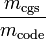$\frac{m_\mathrm{cgs}}{m_\mathrm{code}}$ =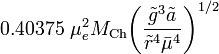$0.40375~\mu_e^2 M_\mathrm{Ch} \biggl( \frac{\tilde{g}^3 \tilde{a}}{\tilde{r}^4 \bar{\mu}^4 } \biggr)^{1/2}$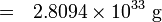$= ~~2.8094\times 10^{33}~\mathrm{g}$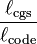$\frac{\ell_\mathrm{cgs}}{\ell_\mathrm{code}}$ =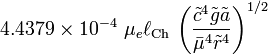$4.4379\times 10^{-4}~ \mu_e \ell_\mathrm{Ch}~\biggl( \frac{\tilde{c}^4 \tilde{g} \tilde{a}} {\bar{\mu}^4 \tilde{r}^4} \biggr)^{1/2}$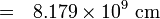$=~~ 8.179\times 10^{9}~\mathrm{cm}$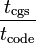$\frac{t_\mathrm{cgs}}{t_\mathrm{code}}$ =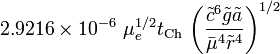$2.9216\times 10^{-6}~\mu_e^{1/2} t_\mathrm{Ch} ~\biggl( \frac{\tilde{c}^6 \tilde{g} \tilde{a}} {\bar{\mu}^4 \tilde{r}^4} \biggr)^{1/2}$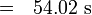$= ~~54.02~\mathrm{s}$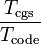$\frac{T_\mathrm{cgs}}{T_\mathrm{code}}$ =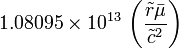$1.08095\times 10^{13} ~\biggl( \frac{\tilde{r} \bar\mu}{\tilde{c}^2} \biggr)$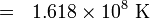$= ~~1.618 \times 10^8~\mathrm{K}$

where: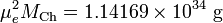$\mu_e^2 M_\mathrm{Ch} = 1.14169\times 10^{34}~\mathrm{g}$;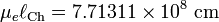$\mu_e \ell_\mathrm{Ch} = 7.71311\times 10^{8}~\mathrm{cm}$;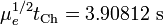$\mu_e^{1/2} t_\mathrm{Ch} = 3.90812~\mathrm{s}$

Case A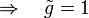$\Rightarrow ~~~\tilde{g} = 1$;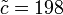$\tilde{c} = 198$;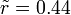$\tilde{r} = 0.44$;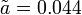$\tilde{a} = 0.044$;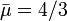$\bar\mu = 4/3$; ρmax = 1;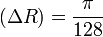$(\Delta R) = \frac{\pi}{128}$

Now let's convert all of the system parameters listed on the accompanying page that details the properties of various polytropic binary systems.

 Properties of (n = 3 / 2) Polytropic Binary Systems Q071 Binary System Accretor Donor q Mtot a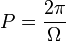$P = \frac{2\pi}{\Omega}$ Jtot Ma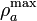$\rho^\mathrm{max}_a$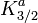$K^a_{3/2}$ Ra Md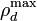$\rho^\mathrm{max}_d$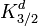$K^d_{3/2}$ Rd fRL SCF units 0.70000 0.02371 0.83938 31.19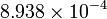$8.938\times 10^{-4}$ 0.013945 1.0000 0.02732 0.2728 0.009761 0.6077 0.02512 0.2888 0.998 conversion2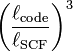$\biggl( \frac{\ell_\mathrm{code}}{\ell_\mathrm{SCF}} \biggr)^3$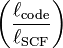$\biggl( \frac{\ell_\mathrm{code}}{\ell_\mathrm{SCF}} \biggr)$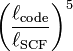$\biggl( \frac{\ell_\mathrm{code}}{\ell_\mathrm{SCF}} \biggr)^5$$\biggl( \frac{\ell_\mathrm{code}}{\ell_\mathrm{SCF}} \biggr)^3$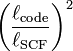$\biggl( \frac{\ell_\mathrm{code}}{\ell_\mathrm{SCF}} \biggr)^2$$\biggl( \frac{\ell_\mathrm{code}}{\ell_\mathrm{SCF}} \biggr)$$\biggl( \frac{\ell_\mathrm{code}}{\ell_\mathrm{SCF}} \biggr)^3$$\biggl( \frac{\ell_\mathrm{code}}{\ell_\mathrm{SCF}} \biggr)^2$$\biggl( \frac{\ell_\mathrm{code}}{\ell_\mathrm{SCF}} \biggr)$ Rad-Hydro units 0.70000 0.6847 2.5752 31.19 0.24293 0.4027 1.0000 0.2571 0.8369 0.28187 0.6077 0.2364 0.88603 0.998 cgs units 0.70000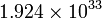$1.924\times 10^{33}$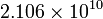$2.106\times 10^{10}$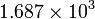$1.687\times 10^{3}$$1.924\times 10^{33}$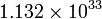$1.132\times 10^{33}$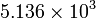$5.136\times 10^{3}$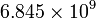$6.845\times 10^{9}$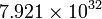$7.921\times 10^{32}$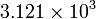$3.121\times 10^{3}$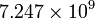$7.247\times 10^{9}$ 0.996 Other units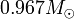$0.967 M_\odot$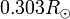$0.303 R_\odot$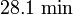$28.1~\mathrm{min}$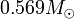$0.569 M_\odot$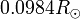$0.0984 R_\odot$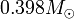$0.398 M_\odot$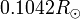$0.1042 R_\odot$ 1Model Q07 (q = 0.700): Drawn from the first page of the accompanying PDF document. NOTE: In this PDF document, Roche-lobe volumes appear to be too large by factor of 2. 2For this model,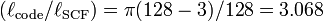$(\ell_\mathrm{code}/\ell_\mathrm{SCF}) = \pi(128 - 3)/128 = 3.068$; see more detailed, accompanying discussion.

Here are some additional useful relations:

General Relation

Case A: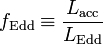$f_\mathrm{Edd} \equiv \frac{L_\mathrm{acc}}{L_\mathrm{Edd}}$ =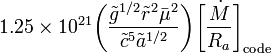$1.25\times 10^{21} \biggl( \frac{\tilde{g}^{1/2} \tilde{r}^2 \bar{\mu}^2 }{\tilde{c}^5 \tilde{a}^{1/2}} \biggr) \biggl[ \frac{\dot{M}}{R_a} \biggr]_\mathrm{code}$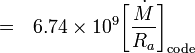$= ~~6.74\times 10^9 \biggl[ \frac{\dot{M}}{R_a} \biggr]_\mathrm{code}$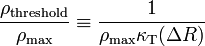$\frac{\rho_\mathrm{threshold}}{\rho_\mathrm{max}} \equiv \frac{1}{\rho_\mathrm{max}\kappa_\mathrm{T} (\Delta R)}$ =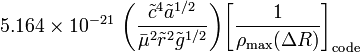$5.164\times 10^{-21}~\biggl( \frac{\tilde{c}^4 \tilde{a}^{1/2}}{\bar{\mu}^2 \tilde{r}^2 \tilde{g}^{1/2}} \biggr) \biggl[ \frac{1}{\rho_\mathrm{max}(\Delta R)} \biggr]_\mathrm{code}$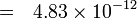$=~~ 4.83\times 10^{-12}$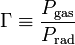$\Gamma \equiv \frac{P_\mathrm{gas}}{P_\mathrm{rad}}$ =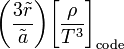$\biggl( \frac{3\tilde{r}}{\tilde{a}} \biggr) \biggl[ \frac{ \rho }{T^3} \biggr]_\mathrm{code}$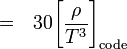$= ~~30 \biggl[ \frac{ \rho }{T^3} \biggr]_\mathrm{code}$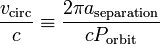$\frac{v_\mathrm{circ}}{c} \equiv \frac{2\pi a_\mathrm{separation}}{c P_\mathrm{orbit}}$ =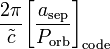$\frac{2\pi}{\tilde{c}} \biggl[\frac{a_\mathrm{sep}}{P_\mathrm{orb}}\biggr]_\mathrm{code}$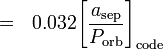$= ~~0.032 \biggl[\frac{a_\mathrm{sep}}{P_\mathrm{orb}}\biggr]_\mathrm{code}$

Case A$\Rightarrow ~~~\tilde{g} = 1$;$\tilde{c} = 198$;$\tilde{r} = 0.44$;$\tilde{a} = 0.044$;$\bar\mu = 4/3$; ρmax = 1;$(\Delta R) = \frac{\pi}{128}$

Combining the above Case A relations with the RadHydro-code properties of the Q0.7 polytropic binary that serves as an initial condition for Dominic's simulations, we conclude the following:

(1)  The system will experience "super-Eddington" accretion (i.e., fEdd > 1) when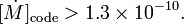$[\dot{M}]_\mathrm{code} > 1.3\times 10^{-10} .$

(2)  The mean-free-path,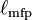$\ell_\mathrm{mfp}$, of a photon will be less than one grid cell R)code when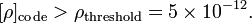$[\rho]_\mathrm{code} > \rho_\mathrm{threshold} = 5\times 10^{-12} .$

(3)  The system is weakly relativistic because,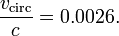$\frac{v_\mathrm{circ}}{c} = 0.0026 .$

 © 2014 - 2020 by Joel E. Tohline |   H_Book Home   |   YouTube   | Appendices: | Equations | Variables | References | Ramblings | Images | myphys.lsu | ADS |